# 10 The Three Ways Grade is Defined

Click play on the following audio player to listen along as you read this section.The picture to the left is a waterfall and as the name suggests the water travels from a high point to a low point. We could say that the water “falls” from the high point to the low point due to the effects of gravity.

If we apply this to the math we’re going through later in this section we would use the term “total fall” and essentially we’d be defining the vertical distance that an object moves.

If an object moves from point A to point B just using a vertical motion (like the waterfall) then there would be no horizontal movement during that time. If you think about any water fall you might have encountered they generally go straight down.

What if it didn’t go down in a straight line but also moved horizontally as well as vertically?

We still have a vertical drop in this case but it just takes a while to get there. We can measure the horizontal distance it takes to get from “A” to “B”. Take a look at the piece of pipe below. It goes horizontal but falls a little during that time.

Combining the vertical distance travelled with the horizontal distance travelled we can calculate what is known as the grade. You will also hear the grade referred to as slope. We will be using grade during this portion of the book but using the word slope generally indicates the same thing.

Take a look at the cast iron drainage pipe again. You can see that it’s grading down as it goes from left to right. Drainage pipe usually contains soil and waste which drains by gravity. If gravity is to do its work then we must have a grade on the pipe. With drainage pipe we generally refer to the slope in inches per foot. The pipe will fall vertically a certain amount on inches (or fraction of an inch) for every foot that it travels horizontally.

Here is another scenario. Have you ever been on a road trip and come across a hill that goes down very steep? The picture below is a road sign that lets drivers know the road is going to grade down at a rate of 7%. Percentage is another way we can define grade.

If we were to go back to our percentage chapter we would say that 7% is like saying 7 out of 100. What this means is that for every 100 feet driven horizontally the road would drop down 7 feet vertically.

We’ve just gone over two of the three ways we define grade in the trades. Any guesses what the third way is?1. inches per foot
2. percentage
3. ?????Now let’s go through each of the three ways to express grade a little more thoroughly.

# Grade Expressed in Inches Per Foot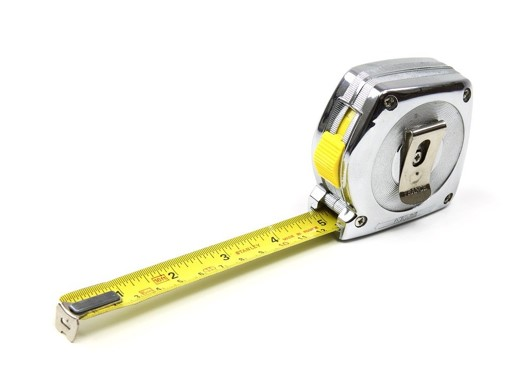Pipe trades workers run pipe requiring grade. As previously stated the waste material running through drainage pipe moves due to gravity and therefore the pipe needs to move both horizontally while at the same time moving vertically. This ensures the smooth movement of the material within the pipe.

Drainage pipe generally runs at ¼ inch per foot. What this means is that for every foot the pipe travels horizontally the pipe falls or drops ¼ inch vertically. This allows the water, soil or waste to move downward simply due to the effects of gravity.

The following are more examples of grades specified in inches per foot.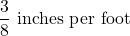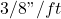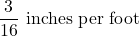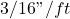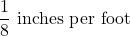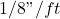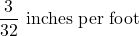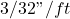In each case, it’s stated that an object is falling vertically at a rate of fractions of an inch for every foot that it travels horizontally. Take a look at the piece of pipe below. Note that in this case it’s running level therefore no grade. We’ll also state that this pipe is one foot long.

Now we’ll grade that pipe at 3/8 of an inch per foot or 3/8”/ft. Take a look at the picture below.

You’ll note the pipe is one foot long but in this case it’s grading down as you go from left to right. During the length of its run it falls (or drops) 3/8 of an inch. This indicates that the grade on the pipe is 3/8”/ft

If you had a 2 foot piece of pipe which graded at 3/8”/ft then it would fall 3/8” for each foot of length. Later on in the chapter we’ll build this into our formula when we start calculating the total fall a pipe has during the length of its run.

One thing to note here is that this type of grade has specific units to it. The grade MUST be expressed in inches per foot.

# Grade Expressed as a ratio

A few chapters back we talked about ratios. An example would be 1 in a 100 or written out it would look like the figure down below.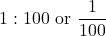In terms of grade we would say that for every 100 units we move horizontally we end up falling one unit. Notice that we’re using the word units but don’t actually specify the units in the grade. When grade is expressed in inches per foot we are attaching units to the grade but when dealing with a ratio there are no specific units.

Although the grade itself does not have specific units we will still be dealing with units of some sort when dealing with the length of a pipe or object. We could refer to all the normal types of units for length we have referenced during this book such as inches, feet, centimeters, meters, yards, miles or any other unit measuring length.

Here are a few examples of grade when dealing with ratios.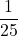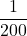Maybe it would be a good idea now to show a visual like we did with inches per foot. Check out the drawing below to see how this works.

What the drawing above is indicating is that for every 50 units of length an object travels horizontally it ends up falling 1 unit vertically. What this tells us is that the grade on the object is 1 in 50. Written as a ratio it would look like the fraction below.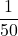We could attach any unit here. If we stated that the object went 50 feet horizontally then we can conclude that the object fell 1 foot during that time. If the object went 50 meters horizontally then it would fall 1 meter.

# Grade Expressed As a Percent

The third way to express grade is as a percent such as 1%, 1.04%, 2% and so on. One of the issues that arises in this case is the manner in which we are shown the grade. If we are told the grade is 2% that won’t actually work for us when we are calculating the total fall later on down the road. As a number it’s fine but to work with it mathematically we have to rework the 2% and get it into a format that is usable.We’ll stay with the example of a 2% grade. If we were to go back to our chapter on percent we would see that saying 2% is like saying 2 out of 100.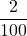You may have noticed that working between a ratio and percent is rather straight forward. Now we could go ahead and use that ratio as our grade. But in this case what we really want to figure out is how we can change the percent to a number we can work with that doesn’t resemble a ratio but its own distinct grade.

Once again visualize that 2% is like 2 out of one hundred.  If we were to take that 2 and divide it by 100 we would end up with 0.02. This is what we are looking for and this is the number we would end up using in our formula when calculating total fall.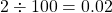Mathematically we take 2% and start with 2 over 100 which ends up as 2 divided by 100 which then goes to 0.02. As stated before 2% is like saying 0.02 when using it in a math formula.

Now instead of going through that on paper or in your mind a much simpler way (assuming you understand the math first) is to take any percentage and move the decimal point two places to the left. Check out the examples below and remember we’re doing this so that later when we are dealing with the total fall formula we have numbers that work in the formula.

Percentages Decimals
2% 0.02
5% 0.05
3% 0.03
1.04% 0.0104
0.76% 0.0076

So a quick recap shows us that there are 3 ways to express grade.